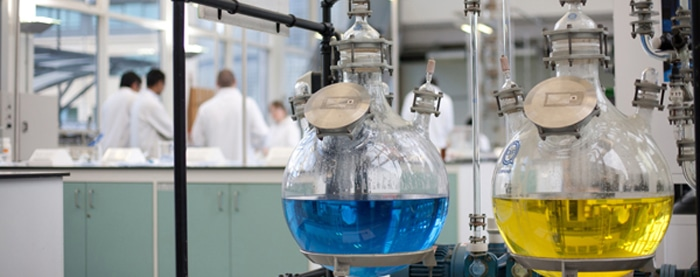Engineering Entrance Sample Papers

Galgotias Engineering entrance exam Chemistry Sample Question PaperQues. The purification of alumina is called
(a) Bosch process
(b) Caster process
(c) Baeyer’s process
(d) Hoop’s process
Ans. (c)

Ques. Which of the following compounds is condensed with Benzaldehyde in Claisen reaction?
(a) Malonic ester
(b) Chloroacetic ester
(c) Acetaldehyde
(d) Acetic anhydride
Ans. (c)

Ques. Capacity of coagulating a colloid by an ion depends on which of the following
(a) Size of the ion
(b) Range of change on the ion
(c) Symbol of charge of the ion
(d) Range as well as sign of charge on the ion
Ans. (d)

Ques. When CO2 is bubbled through a solution of barium peroxide in water
(a) O2 is released
(b) Carbonic acid is formed
(c) H2O2 is formed
(d) No reaction occurs
Ans. (c)

Ques. If 20% nitrogen is present in a compound, its molecular weight will be
(a) 144
(b) 28
(c) 100
(d) 140
Ans. (d)

Ques. Which of the following products is formed on the reaction of acetaldimine and formic acid?
(a) Hydroxamic acid
(b) Acetamide
(c) Ethylamine
(d) Methylamine
Ans. (c)

Ques. Bessemer converter is used for
(a) Steel
(b) Wrought iron
(c) Pig iron
(d) Cast iron
Ans. (c)

Ques. Which of the following is not used in the quantitative estimation of urea?
(a) Urease
(b) NaNO2 + HCl
(c) NaOH + Br2
(d) Oxalic acid
Ans. (d)

Ques. In the presence of 0.025 gram of starch, coagulation ceases on adding 1 ml of 10% NaCl in 10ml gold sol, then gold number of starch will be
(a) 2.5
(b) 25
(c) 0.15
(d) 0.025
Ans. (b)

Ques. Hydroxide soluble in ammonia is
(a) Al(OH)3
(b) Fe(OH)3
(c) Cr(OH)3
(d) Cu(OH)2
Ans. (d)

Ques. Which of the following is the primary reduction product of nitrobenzene?
(a) Hydrazobenzene
(b) p-nitrophenol
(c) Azoxybenzene
(d) N-Phenylhydroxylamine
Ans. (d)

Ques. Upon the addition of a solution A to a strongly acidified solution of barium nitrate, a white precipitate was obtained which did not dissolve even after large addition of water. Solution A contained
(a) Sodium phosphate
(b) Sodium carbonate
(c) Sodium sulphate
(d) Sodium chloride
Ans. (c)

Ques. Density of KCl  is 1.9893 gcm–3 and length of the arm of its unit cell is 6.29082Å, which is determined by X-rays diffraction. What will be the Avogadro number from given data?
(a) 6.023 x 1023
(b) 6.017 x 1023
(c) 6.03 x 1023
(d) 6.017 x 1019
Ans. (b)

Ques. In a compound C, H and N atoms are present in 9 : 1 : 35 by weight. Molecular weight of compound is 108. Molecular formula of compound is
(a) C2H6N2
(b) C3H4N
(c) C6H8N2
(d) C9H12N3
Ans. (c)

Ques. In methane molecule, the hydrogen atoms around carbon  are arranged as
(a) Square planar
(b) Tetrahedral
(c) Triangular
(d) Octahedral
Ans. (b)

Ques. Which of the following reaction is appropriate for knocking out the CO group from the molecule of a carboxylic acid amide?
(a) Rosenmound’s reaction
(b) Cannizzaro’s reaction
(c) Perkin’s reaction
(d) Hofmann’s bromamide reaction
Ans. (d)

Ques. In ethane and cyclohexane which one of the following pairs of conformations are more stable
(a) Eclipsed and chair conformations
(b) Staggered and chair conformations
(c) Staggered and boat conformations
(d) Eclipsed and boat conformations
Ans. (b)

Ques. Oxidising character will be high on high value of
(a) Reduction potential
(b) Oxidation potential
(c) Ionic character
(d) All of the above
Ans. (a)

Ques. The only o, p-directing group which is deactivating in nature is
(a) –NH2
(b) –OH
(c) –X (halogens)
(d) –R (alkyl groups)
Ans. (c)

Ques. Which of the following is a path function as well as an extensive property
(a) Temperature
(b) Intemal energy
(c) Molar heat capacity
(d) Heat capacity
Ans. (d)

Ques. Which of the following names is associated with benzoylation of aniline in the presence of sodium hydroxide ?
(a) Ammonotysis
(b) Acetylation
(c) Carbamoylation
(d) Schotten-Boumann reaction
Ans. (d)

Ques. The outermost shell electronic configuration of alkaline earth metals is
(a) ns2
(b) ns1
(c) np6
(d) nd10
Ans. (a)

Ques. When CO2 dissolves in water, the following equilibrium is established CO2 + 2H2O D H3O+ + HCO3for which the equilibrium constant is 3.8 x 10–7 and pH = 6.0. What would be the ratio of concentration of HCO3to CO2
(a) 3.8 x 10–13
(b) 6.0
(c) 0.38
(d) 13.4
Ans. (c)

Ques. An element of atomic number 29 belongs to
(a) s-block
(b) p-block
(c) d-block
(d) f-block
Ans. (c)

Ques. The lightest metal is
(a) Li
(b) Mg
(c) Ca
(d) Na
Ans. (a)

Ques. The oxidation number of Pt in [Pt(C2H4)Cl3] is
(a) +1
(b) +2
(c) +3
(d) +4
Ans. (b)

Ques. The correct order of increasing C – O bond length of CO, CO32–, CO2 is
(a) CO32– < CO2 < CO
(b) CO2 < CO32– < CO
(c) CO < CO32– < CO2
(d) CO < CO2 < CO32–
Ans. (d)

Ques. One litre of 1 M  CuSO4 solution is electrolyzed. After passing 2F of electricity, molarity of CuSO4 will be
(a) M/2
(b) M/4
(c) M
(d) 0
Ans. (d)

Ques. AlCl3 + Cl D AlCl4,  where Cl is
(a) A Lewis base
(b) A Lewis acid
(c) (a) as well as (b)
(d) None of the above
Ans. (a)

Ques. The product of treating 1-chloro-butane with alcoholic potash would be
(a) 1-butene
(b) 1-butanol
(c) 2-butene
(d) 2-butanol
Ans. (a)